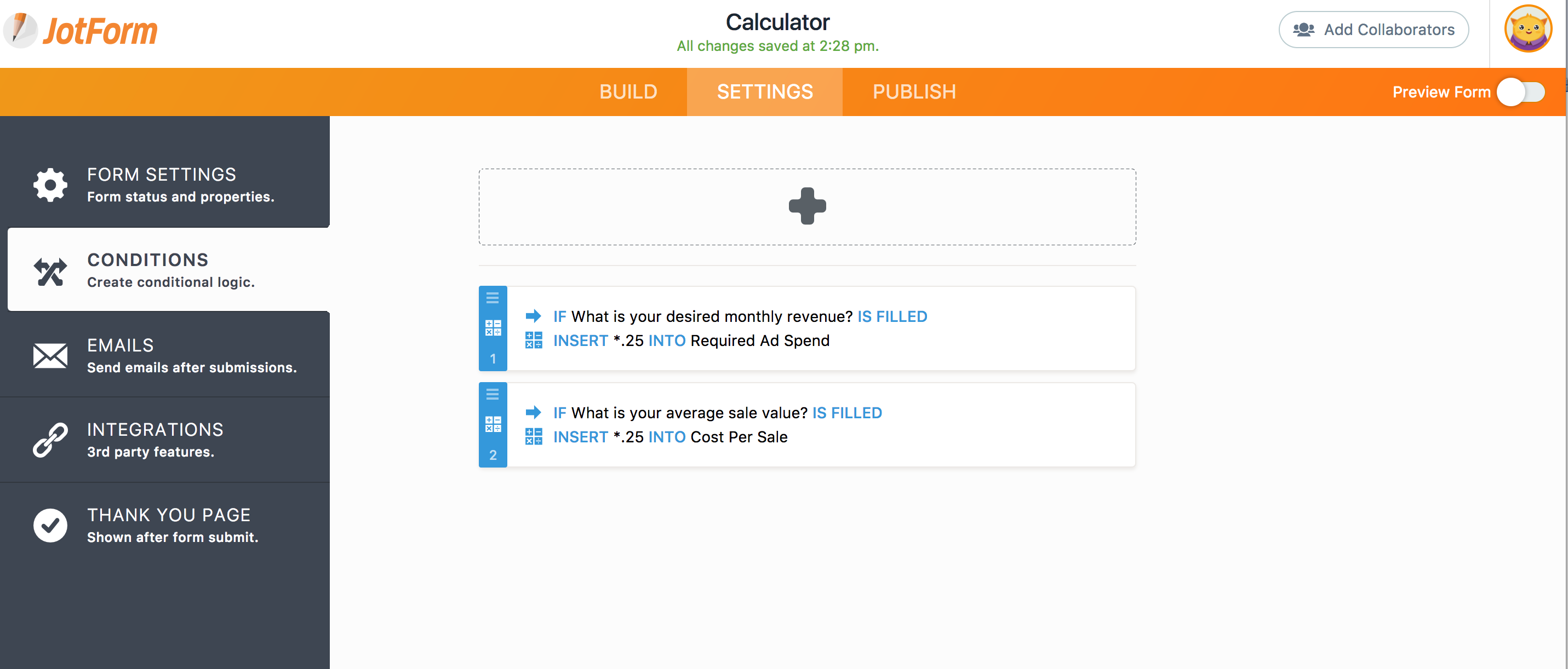Conditional logic not calculating

•tforde
Asked on September 06, 2017 at 01:44 AM
Hi there

Can you help me with why the conditional logic with calculate fields may not be working?

Thanks

Tamra•liyam
Answered on September 06, 2017 at 04:25 AM

I checked your condition for calculation and found it being incomplete. Basically it tries to calculate nothing and multiplies it with .25. Therefore, it won't get any resultWhat you need to do is to add a field by pressing the Add field button and selecting the field where it should be multiplied with. Here is an example:Once you are able to update your conditions, it should run the calculation properly.

If you have questions, please let us know.

•liyam
Answered on September 06, 2017 at 05:34 AM

The problem that I see is you are trying to create your formula out of your product output.A multiplication formula does not work that way. You need two separate values to get a product.

On your calculation, you are getting a value where it is suppose to print the result, which to start with is 0.

I suppose the quick way here is that if you can tell us which fields should be multiplied and where to print that output, it would be great. This way, we can create the formula with you.

Thanks.

•seth
Answered on September 07, 2017 at 02:07 AM

Hello,